• Pytorch 深度学习 模型训练 断点继续训练损失函数恶化或与断点差异较
千次阅读
2019-01-22 03:39:17

# 问题描述

pytorch版本1.0。
神经网络结构主要为残差模型，带有BatchNormalization。
在断点继续训练时，发现模型的训练误差有较大恶化，通常恶化量为10%左右。

# 问题所在

2. 在存储时调用了model的cpu()。想法是合理的，但是cuda()版本的model参数和cpu()版本的参数并不是同一个对象，pytorch理应保持他们的内容一致，但并不是官方的保存model的方法，可能存在bug，因为model中调用的各个子model并不一定就是完全符合官方要求的。那么如何在没有gpu的机子上读取gpu格式的model，在下面会有方法。
3. 模型定义的时候，有时候为了提高代码的复用性，用函数封装了模型，函数则返回模型的对象，这样做是有风险的。笔者就是将各种不同的归一化网络模型封装在一个函数里，通过给予不同参数定义不同的归一化层然后返回他们的对象并构建较大的model，结果则导致了上述问题。这可能是一个pytorch的bug，亦或者pytorch本身就禁止这种用法而笔者孤陋寡闻不知道。

# pytorch模型定义、存储、恢复注意

1. save模型时调用model.state_dict()，optim对象也类似存储方法。
2. load时，torch.load可以规定一个map_loaction=torch.device('cpu') 就可以读取原先以GPU格式保存的数据。
3. 构造optim对象时要先做好模型的cuda()操作。
4. 模型定义的时候不要玩那么多花样，一定使用最简单的，不要用什么函数封装等，确保model中存储子模型的变量是直接由模型对象赋值的。
5. 训练时，每次epoch开始时随机设置一下torch的随机种子，比如torch.manual_seed(numpy.random.randint(1000000))

# 参考过的链接

其他的忘了pytorch 深度学习 checkpoint
更多相关内容
• 如题，训练损失不断增大，测试损失不断减小，两个趋势总是反着的tensorflow 人工智能 深度学习
• self.loss2 = tf.losses.mean_squared_error(reward,pred) self.lr2 = tf.train.exponential_decay(self.lr2_start, self.global_step2, self.lr2_decay_step,self.lr2_decay_rate, staircase=False, name=...tensorflow 机器学习
• 奇怪的事情出现了：训练数据增加，不但没有使机器学习的成绩变好，反而越学越差了。 若将数据增加到30组 outputs = np.random.randint(5, size=(30, 4)) for i in range(30): 修改后的代码如下，另存为...

# 1 发现问题

#加载库
import numpy as np
#生成数据
np.random.seed(0)
outputs = np.random.randint(5, size=(10, 4))
res = []
for i in range(10):
# 假设方程式为 y=4a+6b+7c+2d
y = 4*outputs[i]+6*outputs[i]+7*outputs[i]+2*outputs[i]
res.append([y])
# 定义数据
train_data=np.array(outputs).astype('float32')
y_true = np.array(res).astype('float32')

#定义网络
x = fluid.layers.data(name="x",shape=,dtype='float32')
y = fluid.layers.data(name="y",shape=,dtype='float32')
y_predict = fluid.layers.fc(input=x,size=1,act=None)
#定义损失函数
cost = fluid.layers.square_error_cost(input=y_predict,label=y)
avg_cost = fluid.layers.mean(cost)
#定义优化方法
sgd_optimizer = fluid.optimizer.SGD(learning_rate=0.05)
sgd_optimizer.minimize(avg_cost)
#参数初始化
cpu = fluid.CPUPlace()
exe = fluid.Executor(cpu)
exe.run(fluid.default_startup_program())
##开始训练，迭代500次
for i in range(500):
outs = exe.run(
feed={'x':train_data,'y':y_true},
fetch_list=[y_predict.name,avg_cost.name])
if i%50==0:
print ('iter={:.0f},cost={}'.format(i,outs))
#存储训练结果
params_dirname = "result"
fluid.io.save_inference_model(params_dirname, ['x'], [y_predict], exe)

# 开始预测
infer_exe = fluid.Executor(cpu)
inference_scope = fluid.Scope()
# 加载训练好的模型
with fluid.scope_guard(inference_scope):
[inference_program, feed_target_names,

# 生成测试数据
test = np.array([[,,,]]).astype('float32')
# 进行预测
results = infer_exe.run(inference_program,
feed={"x": test},
fetch_list=fetch_targets)
# 给出题目为 【9,5,2,10】 输出y=4*9+6*5+7*2+10*2的值
print ("9a+5b+2c+10d={}".format(results))


运行结果如下：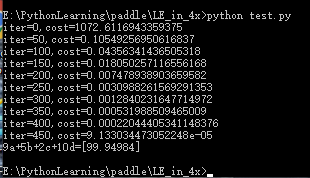学习效果蛮好的。

因官网上要求用fluid.data 代替 fluid.layers.data，故将程序稍作了一点改动：

## 1. 将 fluid.layers.data 改为 fluid.data

### 1.1相应的x的shape改为[-1,4]，y的shape改为[-1,1]

x = fluid.data(name="x",shape=[-1,4],dtype='float32')
y = fluid.data(name="y",shape=[-1,1],dtype='float32')

### 1.2测试数据的shape改为[1,4]

test = np.array([[9,5,2,10]]).astype('float32')

## 2. 想增加模型的准确度，将训练数据由10组增加到20组

outputs = np.random.randint(5, size=(20, 4))
for i in range(20):

修改后的代码如下，另存为 test2.py

#加载库
import numpy as np
#生成数据
np.random.seed(0)
outputs = np.random.randint(5, size=(20, 4))
res = []
for i in range(20):
# 假设方程式为 y=4a+6b+7c+2d
y = 4*outputs[i]+6*outputs[i]+7*outputs[i]+2*outputs[i]
res.append([y])
# 定义数据
train_data=np.array(outputs).astype('float32')
y_true = np.array(res).astype('float32')

#定义网络
x = fluid.data(name="x",shape=[-1,4],dtype='float32')
y = fluid.data(name="y",shape=[-1,1],dtype='float32')
y_predict = fluid.layers.fc(input=x,size=1,act=None)
#定义损失函数
cost = fluid.layers.square_error_cost(input=y_predict,label=y)
avg_cost = fluid.layers.mean(cost)
#定义优化方法
sgd_optimizer = fluid.optimizer.SGD(learning_rate=0.05)
sgd_optimizer.minimize(avg_cost)
#参数初始化
cpu = fluid.CPUPlace()
exe = fluid.Executor(cpu)
exe.run(fluid.default_startup_program())
##开始训练，迭代500次
for i in range(500):
outs = exe.run(
feed={'x':train_data,'y':y_true},
fetch_list=[y_predict.name,avg_cost.name])
if i%50==0:
print ('iter={:.0f},cost={}'.format(i,outs))
#存储训练结果
params_dirname = "result"
fluid.io.save_inference_model(params_dirname, ['x'], [y_predict], exe)

# 开始预测
infer_exe = fluid.Executor(cpu)
inference_scope = fluid.Scope()
# 加载训练好的模型
with fluid.scope_guard(inference_scope):
[inference_program, feed_target_names,

# 生成测试数据
test = np.array([[9,5,2,10]]).astype('float32')
# 进行预测
results = infer_exe.run(inference_program,
feed={"x": test},
fetch_list=fetch_targets)
# 给出题目为 【9,5,2,10】 输出y=4*9+6*5+7*2+10*2的值
print ("9a+5b+2c+10d={}".format(results))


运行结果如下：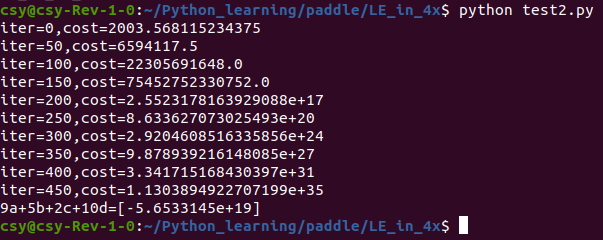奇怪的事情出现了：训练数据增加，不但没有使机器学习的成绩变好，反而越学越差了。

若将数据增加到30组

outputs = np.random.randint(5, size=(30, 4))
for i in range(30):

修改后的代码如下，另存为test3.py

#加载库
import numpy as np
#生成数据
np.random.seed(0)
outputs = np.random.randint(5, size=(30, 4))
res = []
for i in range(30):
# 假设方程式为 y=4a+6b+7c+2d
y = 4*outputs[i]+6*outputs[i]+7*outputs[i]+2*outputs[i]
res.append([y])
# 定义数据
train_data=np.array(outputs).astype('float32')
y_true = np.array(res).astype('float32')

#定义网络
x = fluid.data(name="x",shape=[-1,4],dtype='float32')
y = fluid.data(name="y",shape=[-1,1],dtype='float32')
y_predict = fluid.layers.fc(input=x,size=1,act=None)
#定义损失函数
cost = fluid.layers.square_error_cost(input=y_predict,label=y)
avg_cost = fluid.layers.mean(cost)
#定义优化方法
sgd_optimizer = fluid.optimizer.SGD(learning_rate=0.05)
sgd_optimizer.minimize(avg_cost)
#参数初始化
cpu = fluid.CPUPlace()
exe = fluid.Executor(cpu)
exe.run(fluid.default_startup_program())
##开始训练，迭代500次
for i in range(500):
outs = exe.run(
feed={'x':train_data,'y':y_true},
fetch_list=[y_predict.name,avg_cost.name])
if i%50==0:
print ('iter={:.0f},cost={}'.format(i,outs))
#存储训练结果
params_dirname = "result"
fluid.io.save_inference_model(params_dirname, ['x'], [y_predict], exe)

# 开始预测
infer_exe = fluid.Executor(cpu)
inference_scope = fluid.Scope()
# 加载训练好的模型
with fluid.scope_guard(inference_scope):
[inference_program, feed_target_names,

# 生成测试数据
test = np.array([[9,5,2,10]]).astype('float32')
# 进行预测
results = infer_exe.run(inference_program,
feed={"x": test},
fetch_list=fetch_targets)
# 给出题目为 【9,5,2,10】 输出y=4*9+6*5+7*2+10*2的值
print ("9a+5b+2c+10d={}".format(results))


运行结果如下：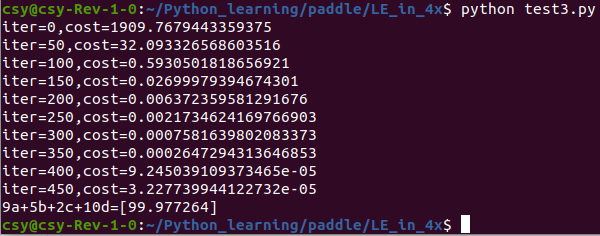哇！学习成绩又提高了。比10组数据训练还要好。

# 2 查找问题

为了查找问题，对程序进行了一些修改和简化：

1.改为用键盘输入数字确定组数，生成训练数据
2.改为用键盘输入数字确定生成的训练数据范围
3.改为用键盘输入数字确定随机种子
5.随机产生浮点数的训练数据
6.打印出训练数据
7.不保存模型
8.删除测试部分

修改后的代码如下，另存为test7.py

#加载库
import numpy as np

#生成数据
group = input('请输入一个正整数确定生成数据的数量：')
group = int(group)
data_range = input('请输入一个正整数确定生成训练数据的取值范围：')
float_range = float(data_range)
random_seed = input('请输入一个正整数作为随机数的种子：')

np.random.seed(int(random_seed))
outputs =np.random.uniform(1,float_range,size=(group,4)) #float64

res = []  #生成一个空list
for i in range(group):
#假设方程式为 y=4a+6b+7c+2d，生成答案
y = 4*outputs[i]+6*outputs[i]+7*outputs[i]+2*outputs[i]
print("{}: {}=({},{},{},{})".format(i,y,outputs[i],outputs[i],outputs[i],outputs[i]))
res.append([y])  # 当变量为array[][]时，对应的y值保存在res

# 定义数据
train_data = np.array(outputs).astype('float32')   # 训练数据使用10组随机生成的数据，将整型随机数改为浮点型
y_true = np.array(res).astype('float32')           # 对应的标准答案
print(train_data.shape,y_true.shape)

# 定义网络
x = fluid.data(name="x",shape=[None,4],dtype='float32')
y = fluid.data(name="y",shape=[None,1],dtype='float32')
y_predict = fluid.layers.fc(input=x,size=1,act=None)

# 定义损失函数
cost = fluid.layers.square_error_cost(input=y_predict,label=y)
avg_cost = fluid.layers.mean(cost)

# 定义优化
sgd_optimizer = fluid.optimizer.SGD(learning_rate=0.05)
sgd_optimizer.minimize(avg_cost)

#参数初始化
cpu = fluid.CPUPlace()
exe = fluid.Executor(cpu)
exe.run(fluid.default_startup_program())

## 开始训练，迭代500次
for i in range(500):
outs = exe.run(
feed={'x':train_data,'y':y_true},
fetch_list=[y_predict.name,avg_cost.name])
if i%50==0:
print('iter={:.0f},cost={}'.format(i,outs))


第一次运行：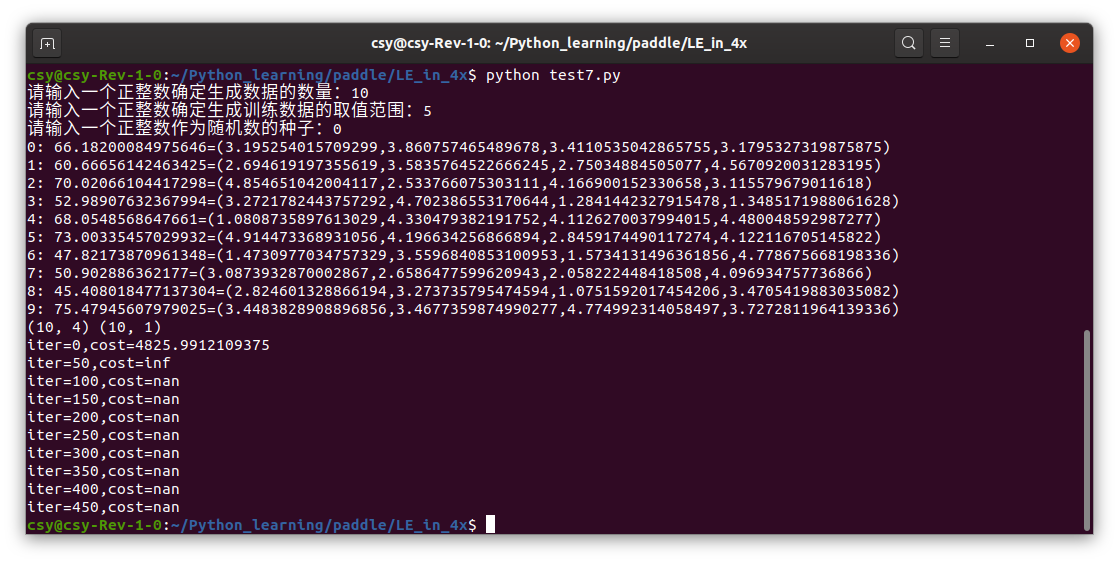第二次运行：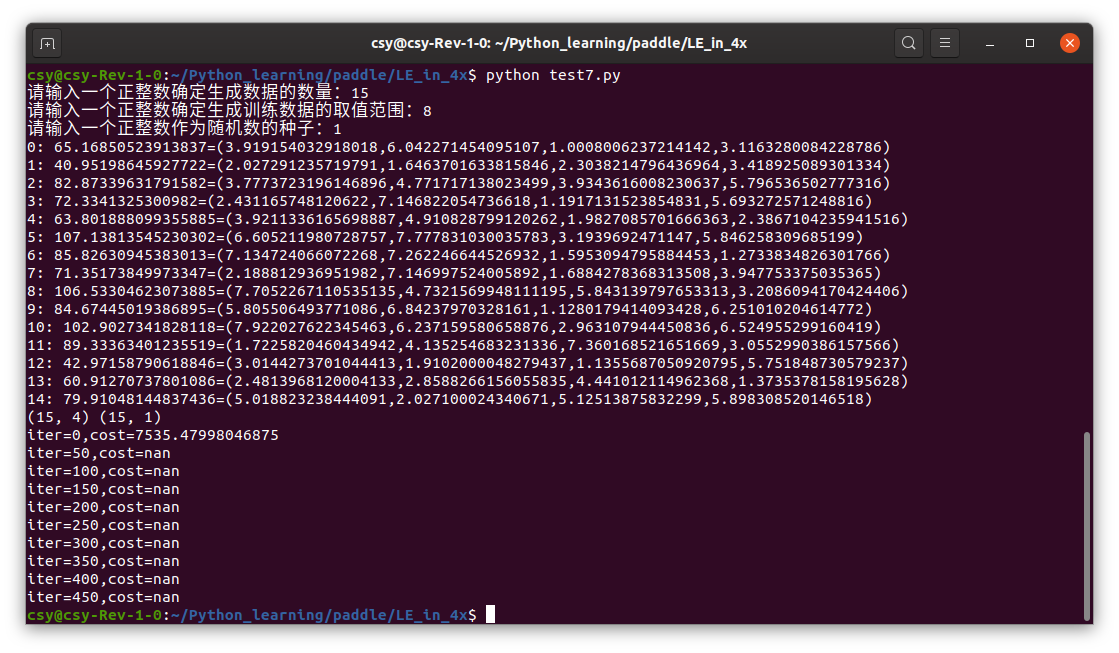将学习率改为0.01

sgd_optimizer = fluid.optimizer.SGD(learning_rate=0.01)

运行结果：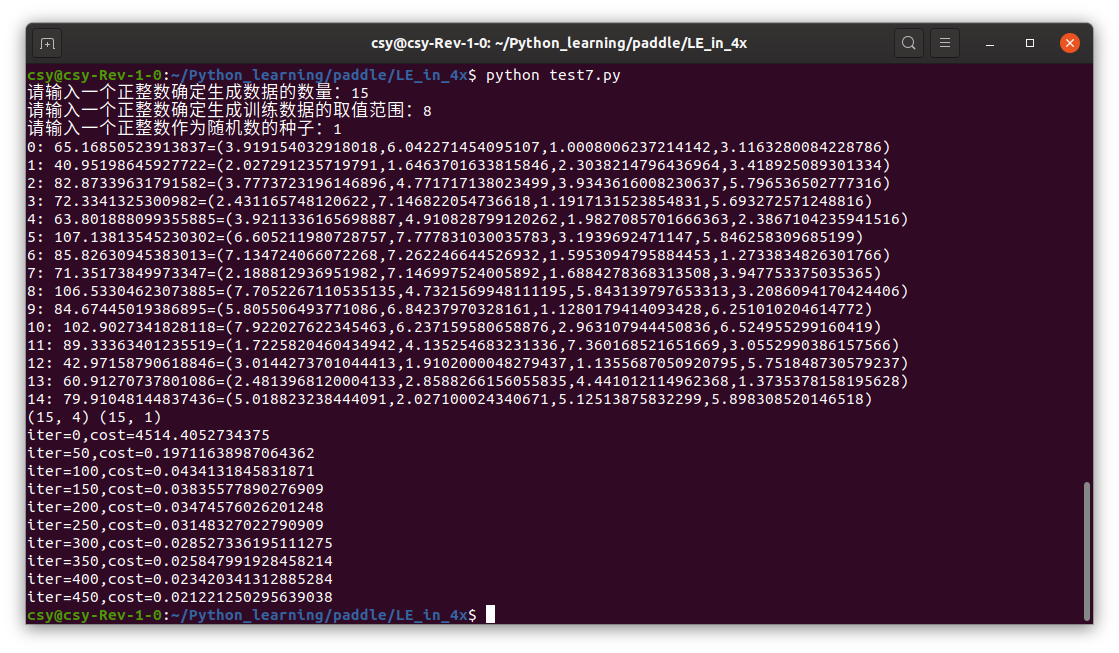再将test2.py的学习率修改为0.01，运行结果：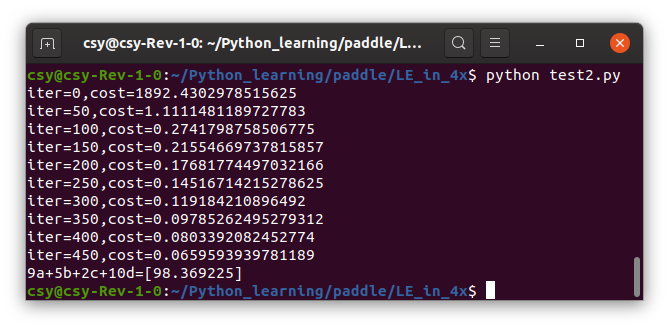学习率降低后，每次训练都有进步，只是进步不大。但不会退步。

# 3 我的结论

训练数据的shape和type，必须和fluid.data的shape和type一致。训练数据的shape和type，也必须和fluid.data的shape和type一致。

学习率高，相当于学生跳级。顺利的话，比别的学生学的快，早毕业。但是也可能不如按部就班的学生有进步，也就是有拔苗助长的风险。学习率低，可以做到天天向上。要在稳健和速度上进行调整。

训练的数据范围最好要涵盖测试数据的范围。考试题如果是老师上课讲过的，考试成绩就会好一些。

训练数据的数量越大，损失函数的值越漂亮。如果相反，则应考虑学习率是否需要调低一些。

对于学习率低的网络，可以采取笨鸟先飞的办法，多迭代几次来弥补进步慢的不足。当然也要警惕迭代过多可能引起过拟合。

展开全文paddle 线性回归 学习率
• 训练模型第一阶段过程当中，我们是使用有标签样本进行训练，在线性回归当中我们也可以看到，有很多点并不是直接通过了直线，让我们来看一下这张数据图表。 线性回归 我们可以看到，有一些点是没有通过直线的...

在训练模型第一阶段过程当中，我们是使用有标签样本进行训练，在线性回归当中我们也可以看到，有很多点并不是直接通过了直线，让我们来看一下这张数据图表。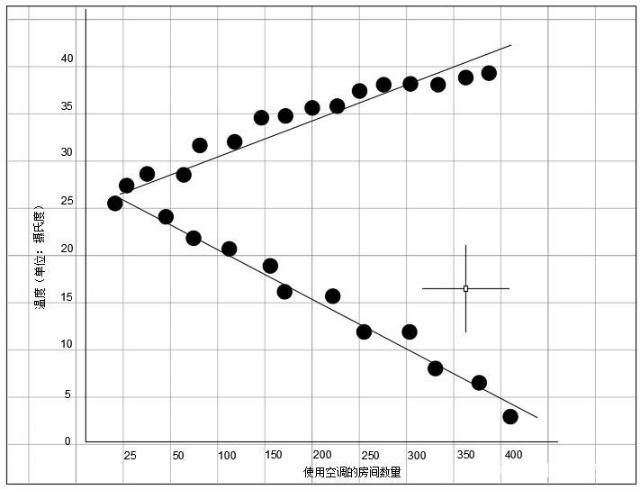线性回归

我们可以看到，有一些点是没有通过直线的，机器学习的算法是通过检查多个样本并尝试找出最大限度地减少损失的模型。这一过程我们称之为经验风险最小化。损失是一个值，表示对单个样本而言模型预测的准确程度。如果模型预测的完全准确，那么损失就为零，否则损失就会较大。训练模型的目标是从所有的样本中找到一组平均损失较小的“权重”和“偏差”。让我们来看一下下面的图，红色表示损失，蓝色表示预测。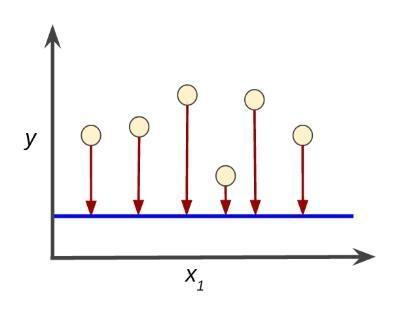图1 - 损失较大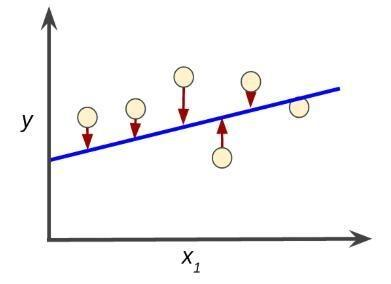图2 - 损失较小

图1中红色的的线是比较长的，所以这种损失就会比较大。因为图1中预测的线几乎没有寻找样本的规律，所以我们需要一种创建一种数学函数，以有意义的方式来汇总各个损失，这种函数我们称之为损失函数。

平方损失

线性回归模型中，我们使用的就是平方损失（又称L2损失）的损失函数。我们来看一下单个样本的平方损失代码；

= the square of the difference between the label and the prediction

= (observation - prediction(x))^2（^2是2次方的意思）

= (y - y')2

均方误差（MSE）

均方误差指的是每个样本的平均平方损失。要计算均方误差，就要求出所有样本的各个平方损失之和，然后我们再除以样本数量。公式如下：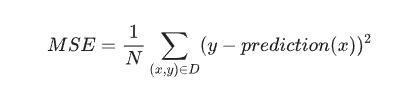均方误差公式

（x，y）指的是样本。x指的是模型进行预测中使用的特征数据集（如：使用空调数量）；y指的是样本的标签（如：室外的温度）prediction（x）指的是权重和偏差与特征x结合的函数D指的是所有有标签样本的数据集N指的是D中的样本数量

展开全文• IMG_W = 64 # resize图像，太的话训练时间久 IMG_H = 64 BATCH_SIZE = 20 CAPACITY = 200 MAX_STEP = 10000 # 一般大于10K learning_rate = 0.00005 # 一般小于0.0001 # 获取批次batch train_dir = 'D:/...人工智能 神经网络 深度学习
• 听了李宏毅老师的深度学习的课程里面关于梯度下降算法的理解，才开始去真正理解一个网络的损失函数及优化过程，思考可能不够全面，希望补充交流。 梯度下降算法用于神经网络参数的更新，使得网络能够拟合出合适的...

听了李宏毅老师的深度学习的课程里面关于梯度下降算法的理解，才开始去真正理解一个网络的损失函数及优化过程，思考可能不够全面，希望补充交流。

梯度下降算法用于神经网络参数的更新，使得网络能够拟合出合适的参数用于解决一类具体的问题，比如图像分类或者是图像分割等。

传统的梯度下降算法：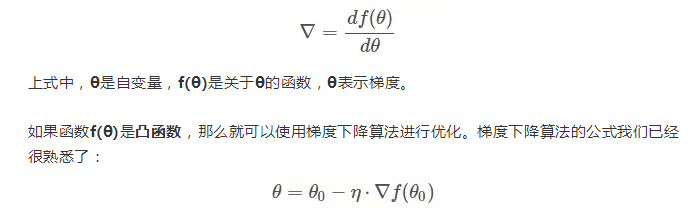其中，θo是自变量参数，即下山位置坐标，η是学习因子，即下山每次前进的一小步（步进长度），θ是更新后的θo，即下山移动一小步之后的位置。

这里是将梯度下降比作从一座山下来寻找最低点。f是损失函数，损失函数是网络输出值和预期之间的差值，在监督学习过程中，预期是数据的标签。因为损失函数实际包含网络输出值，网络输出值由网络参数计算得到，故通过对损失函数可以实现对参数的更新，下面介绍梯度下降法的数学原理。

推导过程使用到泰勒级数：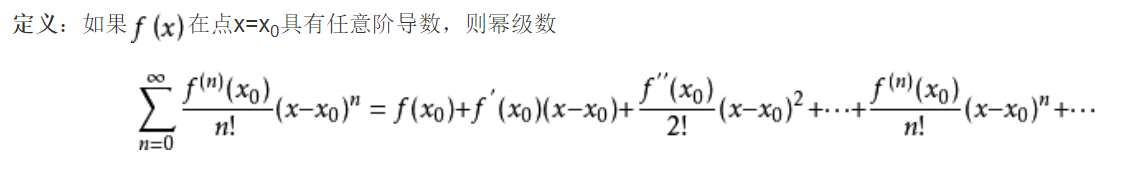当x趋近x0时，泰勒级数变为：f(x) = f(x0) + f ' (x0)(x-x0)

将里面的参数替换成损失函数的参数：f(θ) = f(θo) + f '(θ)(θ-θo)

f(θo) 相当于是参数在θo的具体值，通过现在的参数值和损失函数的梯度来实现参数更新。此时需要保证的是两个参数是趋近的，不然梯度下降法不成立。在 f '(θ)(θ-θo) 前面加一个常数表示学习率，来进一步控制参数的变化量，使得更新的参数不会在损失函数的最小值周围震荡。

学了梯度下降算法的原理之后，思考非监督学习的网络是怎么训练的？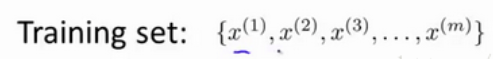在这里我们有一系列点，却没有标签。

非监督学习是希望通过算法去寻找数据的内在结构。这里介绍简单的非监督学习的算法，聚类算法。简单来说，聚类算法就是将数据分为点集（簇）。

K-均值算法:
K-均值是最普及的聚类算法，算法接受一个未标记的数据集，然后将数据聚类成不同的组。

K-均值是一个迭代算法，假设我们想要将数据聚类成n个组，其方法为:

首先选择K个随机的点，称为聚类中心（cluster centroids）；

聚类算法会做两件事：
1.簇分配
2.移动聚类中心

对于数据集中的每一个数据，按照距离K个中心点的距离，将其与距离最近的中心点关联起来，与同一个中心点关联的所有点聚成一类。

计算每一个组的平均值，将该组所关联的中心点移动到平均值的位置。

聚类算法的损失函数是数据到聚类中心的据离。聚类算法的思想是：

(1)选定聚类中心，计算数据到各个聚类中心的距离，选择距离最近的聚类中心作为数据的临时索引。

然后给数据分配临时的索引，通过改变数据的索引来最小化数据到聚类中心的距离（最小化损失函数）。

(2)改变聚类中心。计算数据到聚类中心的距离，最小化距离寻找新的聚类中心。

所以聚类算法需要更新的参数是数据的索引值和聚类中心，直到所有数据到各自的聚类中心的距离最小时，完成迭代。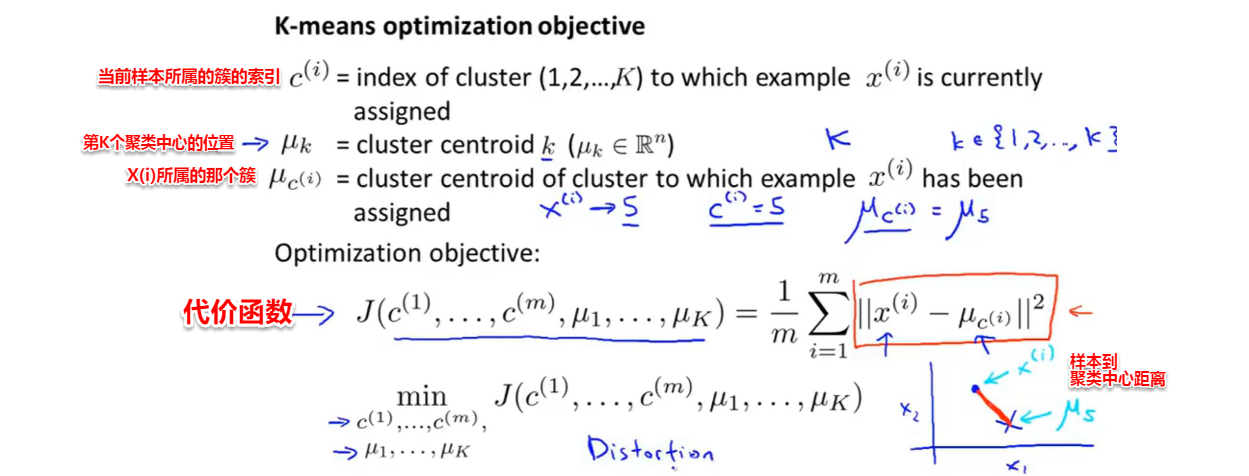聚类算法的优化参数是数据的临时索引和聚类中心，最终的优化目标是使数据到聚类中心的距离最小。

展开全文深度学习神经网络 机器学习 深度学习
• 在代码中添加以下两行可以解决： torch.backends.cudnn.enabled = True torch.backends.cudnn.benchmark = True 原文：https://www.jianshu.com/p/8486076ddd10深度学习 tensorflow pytorch caffe 机器学习
• 今天小编就为大家分享一篇Tensorflow训练模型越来越慢的2种解决方案，具有很好的参考价值，希望对大家有所帮助。一起跟随小编过来看看吧 1 解决方案 【方案一】 载入模型结构放在全局，即tensorflow会话外层。 '''...python 人工智能 tensorflow 深度学习
• 1 解决方案 【方案一】 载入模型结构放在全局，即tensorflow会话外层。 '''载入模型结构:最关键的一步''' saver = tf.train.Saver() ... '''开始训练''' _, loss_1, acc, summary = sess.run(...tensorflow
• 训练神经网络过程中，经过softmax函数输出概率后，当当前最大概率对应的类别与训练标签一致时，损失Loss往往较小，而经过softmax输出时，训练标签所对应的概率softmax概率较小，此时的Loss计算结果往往很。...前向传播 Loss计算
• 最近上手pytorch，搭了一个3D版本的Alexnet模型同于对医学图像简单的进行分类，期间又踩了一个坑，损失函数的loss值在第二步就飙到了一万多，查了好几天也没查到为啥，最初是以为图像预处理部分的问题，（图像归一化...深度学习
• 自定义损失函数：函数定义、类定义（用得更多）。注意涉及到数学运算时，我们最好全程使用PyTorch提供的张量计算接口，这样就不需要我们实现自动求导功能并且我们可以直接调用cuda。 动态调整学习率：pytorch内置...pytorch 深度学习
• 如下图：（值到吐血） 于是乎，开始心酸找错历程（暴哭） 解决过程 1.通常先查看数据集。图像和标签是否对应。没问题（√） 2.模型结构问题，特别是最后输出时种类个数对不对。没问题（√） 3.权重初始化...深度学习 keras深度学习深度学习 机器学习 人工智能 python 神经网络
• 现在的模型越来越大训练样本越来越多，每个样本都需要经过模型的全部计算，这就导致了训练成本的平方级增长。为了解决这个问题，即将大模型拆分成多个小模型，对于一个样本来说，无需经过所有的小模型去计算，而...深度学习
• 不同的模型有不同的计算量，当需要的计算量很时，耗时也会很 权重初始化问题。常用的初始化方案有全零初始化、正态分布初始化和均匀分布初始化等，合适的初始化方案很重要，之前提到过神经网络初始化为0可能会...神经网络 深度学习 机器学习
• https://blog.csdn.net/u013249853/article/details/89393982
• DL之AlexNet：AlexNet算法的架构详解、损失函数、网络训练和学习之详细攻略 相关文章Dataset：数据集集合(CV方向数据集)——常见的计算机视觉图像数据集集合(建议收藏，持续更新)DL之CNN(paper)：关于CNN...
• Wasserstein GAN（带梯度罚分） Keras模型和张量流优化 ... 它会产生看起来很伪造的图像，但损失（产生器和鉴别器）在学习过程中会越来越大。 ！ 因此它可能有一些不完整的要点，希望有人检查一下，谢谢。
• 训练过程中，loss的值非常，可能是： （1）没有除以归一化的因子（GAN中需要除以256*256*3），或者没有取平均值 （2）learning rate太，导致更新之后的权值参数特别，从而下一次计算prediction的时候数值...
• 一种训练稀疏网络的方法，不需要重训练，一次完成，又快速，又不损失性能，边缘设备部署必备。 这篇博客文章是关于我和 Luke Zettlemoyer 的工作， Sparse Networks from Scratch: Faster Training without Losing ...
• 平方误差和交叉熵是神经网络中很常用的损失函数，那么平方误差和交叉熵...接下来我们使用平方误差损失训练这个神经元，接受输入1.0，目标输出0.0。首先将权重初始化为0.6，偏置0.9，学习率为0.15，我们来看看损失...
• 介绍如何使用PyTorch自带的amp模块进行混合精度训练
• ## DDPG训练问题

千次阅读 2020-11-01 11:20:56
target)-Q)^2)，actor网络的损失函数是Q，critic网络的参数更新公式是Wq=Wq-αdQ/dWq，critic网络的参数更新公式是uWu=Wu-β*(dQ/da*du/dWu)，根据以上参数更新公式进行训练时，Q值是递减的，损失函数的变化也与奖励...
• 对于刚开始学习深度学习的人，可能都踩过这样的坑，即该次acc或loss与上次训练结果差距较，或者说前面几次收敛了而本次没怎么收敛。造成这种结果的原因是什么呢？ 了解cnn网络或者mlp的小伙伴都知道，权值在每次...深度学习 人工智能 tensorflow python
• LSTM训练时出现梯度爆炸 训练过程中出现loss突然变几十倍的情况，再慢慢减小，再突然变。即梯度爆炸。 更改 activation=‘sigmoid’ 激活函数 RNN梯度消失梯度爆炸 ...机器学习
• 全网最全的超大模型和分布式训练架构梳理，并附上经典论文简介。分布式 架构 深度学习 人工智能...